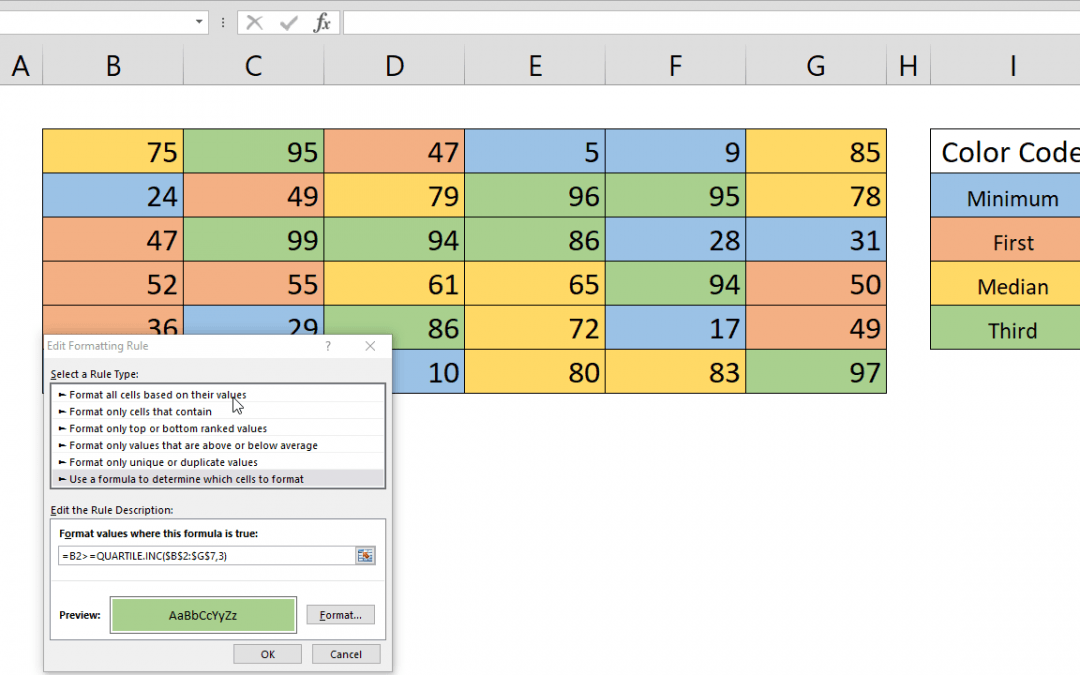Select Page## How to highlight quartiles in Excel

It is often a good idea to highlight duplicate values in a data set to help easily identify the outliers. In this article we are going to explain how to find quartiles in Excel and highlight them dynamically with Conditional Formatting. Syntax = relative reference of...## How to create a Quarter formula in Excel

There is no specific Quarter formula in Excel. But an Excel quarter formula can be constructed by combining ROUNDUP and MONTH functions with custom number formatting. Syntax =ROUNDUP( MONTH( date value ) /3, 0 ) Steps Start with =ROUNDUP( function Continue with MONTH(...## How to generate Excel month names

This article shows you how to generate month list by formulas and how to display Excel month names instead of actual date values. We will use DATE, YEAR and MONTH formulas as well as Number Formatting. Syntax =DATE( YEAR( previous date ), MONTH( previous date )+1, 1)...## How to calculate Excel Table totals

This article shows how to calculate Excel table totals by using Excel Table which is one of the most underrated features in Excel. Steps Select a cell inside your table or whole range Press Ctrl + T or click Table icon under INSERT tab on the ribbon Make sure My table...## How to use Excel sort function

Although Excel has a built-in sort feature, there are times you may need to sort data by formula or in a formula. This article shows how to use Excel sort function with SMALL and ROW functions. Syntax =SMALL( column of list, ROW()-ROW(row of list’s title)) Steps...## How to create Excel data validation for unique values

Entering data into an Excel file manually carries the risk of incorrect entries. Using Excel data validation feature reduces and in many cases complete eliminates this risk. In this article, we are going to show how to combine COUNTIF function with Data Validation...## How to create a dynamic drop down list in Excel

Creating a dynamic Excel drop down list is very useful If you have a list that is updated frequently. This article shows you how to create a dynamic drop down list with the help of OFFSET and COUNTA functions. Syntax =OFFSET(title of list, 1, 0, COUNTA(column that...## How to get Excel cell address of a lookup result

This article shows you how to get Excel cell address of a lookup result by using the CELL and INDEX functions. Syntax =CELL( “address”, INDEX( data, MATCH( lookup value for row, lookup range for row, 0 ), column index ) ) Steps Start with the =CELL(...## How to change number format in Excel chart

Excel charts are one of the most used and easy to understand data visualization tools. Excel provides many chart types as well as numerous personalization options. One of the cool features is the ability to change number format in Excel chart. Steps Double-click on...